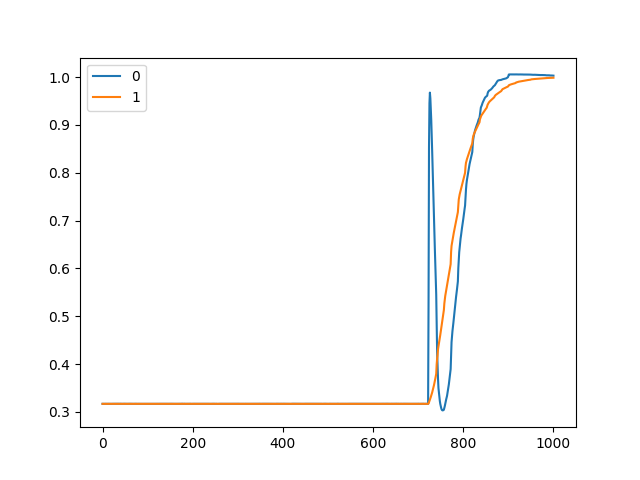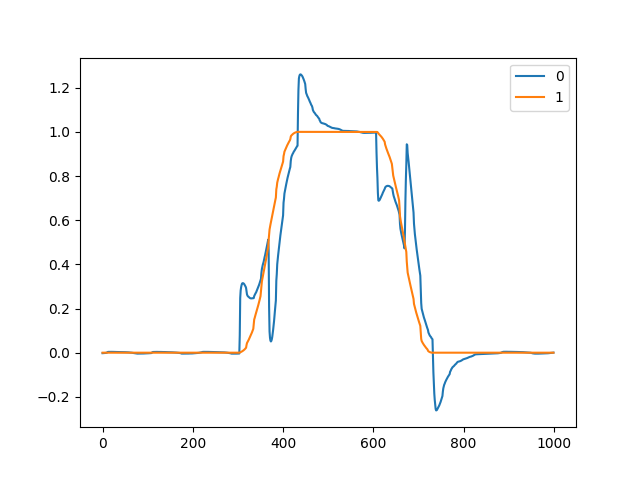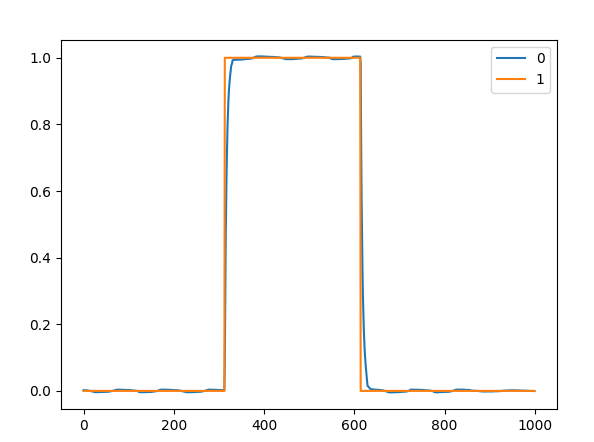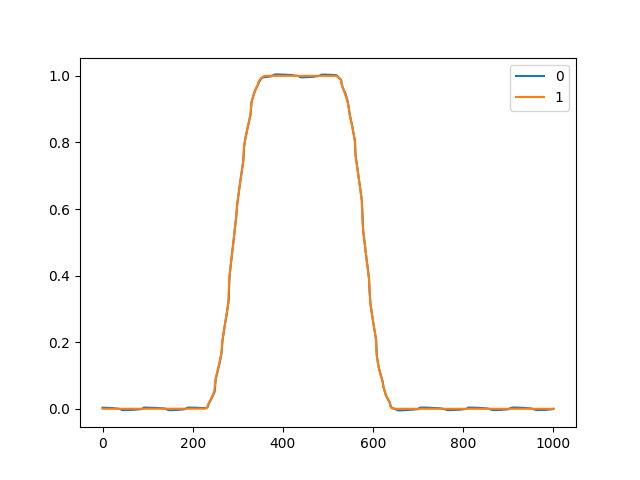# My motor won't move, without any errors

Hi, this is my first post, but I’m struggling to figure out how can I have drive my BLDC via ODrive motor driver.

I think I wired up successfully, and setup my driver by odrive-tools.
I’m sure there’re no errors, and my encoder works correctly, and the position can be monitored by live-plotter when I rotate my motor by my hand.

Along the document, I set up the driver and successfully completed calibration by typing (it beeps and rotates for both direction):
`odrv0.axis0.requested_state = AXIS_STATE_FULL_CALIBRATION_SEQUENCE`

But after that, I can’t feel any brake or holding force, though I can feel very very weak vibration, after when I type :
`odrv0.axis0.requested_state = AXIS_STATE_CLOSED_LOOP_CONTROL`

And I can’t drive my motor by any CONTROL_MODE, position, torque, nor velocity, either.
My command is like:
`odrv0.axis0.controller.config.input_mode = CONTROL_MODE_POSITION_CONTROL`
`In : odrv0.axis0.controller.input_pos = 1`
`In : odrv0.axis0.controller.input_pos = 0.1`

The motor wouldn’t move!

I hope someone can help me…
Here’s my environment and odrive-tools status:

OS:Ubuntu18.04
odrive-tool version : ODrive control utility v0.5.1.post0 (I’m sure I updated it by “odrivetool dfu”)
My motor(type is KV280) : https://ja.aliexpress.com/item/32729435818.html?spm=a2g0s.9042311.0.0.7b154c4dIjUOQV
My encoder : https://www.digikey.jp/product-detail/ja/ams/AS5048A-TS_EK_AB/AS5048A-AB-1.0-ND/3188612

In : dump_errors(odrv0)
axis0
axis: no error
motor: no error
fet_thermistor: no error
motor_thermistor: no error
encoder: no error
controller: no error
axis1
axis: no error
motor: no error
fet_thermistor: no error
motor_thermistor: no error
encoder: no error
controller: no error

In : odrv0.axis0.motor.config
Out:
pre_calibrated = False (bool)
pole_pairs = 7 (int)
calibration_current = 5.0 (float)
resistance_calib_max_voltage = 2.0 (float)
phase_inductance = 2.8769496566383168e-05 (float)
phase_resistance = 0.0807584673166275 (float)
torque_constant = 0.02953571453690529 (float)
direction = 1 (int)
motor_type = 0 (int)
current_lim = 20.0 (float)
current_lim_margin = 8.0 (float)
torque_lim = inf (float)
inverter_temp_limit_lower = 100.0 (float)
inverter_temp_limit_upper = 120.0 (float)
requested_current_range = 60.0 (float)
current_control_bandwidth = 1000.0 (float)
acim_slip_velocity = 14.706000328063965 (float)
acim_gain_min_flux = 10.0 (float)
acim_autoflux_min_Id = 10.0 (float)
acim_autoflux_enable = False (bool)
acim_autoflux_attack_gain = 10.0 (float)
acim_autoflux_decay_gain = 1.0 (float)

In : odrv0.axis0.encoder.config
Out:
mode = 257 (int)
use_index = False (bool)
find_idx_on_lockin_only = False (bool)
abs_spi_cs_gpio_pin = 4 (int)
zero_count_on_find_idx = True (bool)
cpr = 16384 (int)
offset = 18950 (int)
pre_calibrated = False (bool)
offset_float = 1.0753750801086426 (float)
enable_phase_interpolation = True (bool)
bandwidth = 1000.0 (float)
calib_range = 0.019999999552965164 (float)
calib_scan_distance = 50.26548385620117 (float)
calib_scan_omega = 12.566370964050293 (float)
idx_search_unidirectional = False (bool)
ignore_illegal_hall_state = False (bool)
sincos_gpio_pin_sin = 3 (int)
sincos_gpio_pin_cos = 4 (int)

In : odrv0.axis0.config
Out:
startup_motor_calibration = False (bool)
startup_encoder_index_search = False (bool)
startup_encoder_offset_calibration = False (bool)
startup_closed_loop_control = False (bool)
startup_sensorless_control = False (bool)
startup_homing = False (bool)
enable_step_dir = False (bool)
step_dir_always_on = False (bool)
turns_per_step = 0.0009765625 (float)
watchdog_timeout = 0.0 (float)
enable_watchdog = False (bool)
step_gpio_pin = 1 (int)
dir_gpio_pin = 2 (int)
calibration_lockin:
current = 10.0 (float)
ramp_time = 0.4000000059604645 (float)
ramp_distance = 3.1415927410125732 (float)
accel = 20.0 (float)
vel = 40.0 (float)
sensorless_ramp:
current = 10.0 (float)
ramp_time = 0.4000000059604645 (float)
ramp_distance = 3.1415927410125732 (float)
accel = 200.0 (float)
vel = 400.0 (float)
finish_distance = 100.0 (float)
finish_on_vel = True (bool)
finish_on_distance = False (bool)
finish_on_enc_idx = False (bool)
general_lockin:
current = 10.0 (float)
ramp_time = 0.4000000059604645 (float)
ramp_distance = 3.1415927410125732 (float)
accel = 20.0 (float)
vel = 40.0 (float)
finish_distance = 100.0 (float)
finish_on_vel = False (bool)
finish_on_distance = False (bool)
finish_on_enc_idx = False (bool)
can_node_id = 0 (int)
can_node_id_extended = False (bool)
can_heartbeat_rate_ms = 100 (int)

In : odrv0.axis0.controller.config
Out:
gain_scheduling_width = 10.0 (float)
enable_vel_limit = True (bool)
enable_current_mode_vel_limit = False (bool)
enable_gain_scheduling = False (bool)
enable_overspeed_error = True (bool)
control_mode = 1 (int)
input_mode = 3 (int)
pos_gain = 20.0 (float)
vel_gain = 0.1599999964237213 (float)
vel_integrator_gain = 0.3199999928474426 (float)
vel_limit = 1000.0 (float)
vel_limit_tolerance = 1.2000000476837158 (float)
vel_ramp_rate = 10000.0 (float)
torque_ramp_rate = 0.009999999776482582 (float)
circular_setpoints = False (bool)
circular_setpoint_range = 1.0 (float)
homing_speed = 0.25 (float)
inertia = 1.0 (float)
axis_to_mirror = 255 (int)
mirror_ratio = 1.0 (float)
input_filter_bandwidth = 2.0 (float)
anticogging:
index = 0 (int)
pre_calibrated = False (bool)
calib_anticogging = False (bool)
calib_pos_threshold = 1.0 (float)
calib_vel_threshold = 1.0 (float)
cogging_ratio = 1.0 (float)
anticogging_enabled = True (bool)

`control_mode = 1`
`input_mode = 3`

You’re in torque control mode and position_filter input mode. I think you meant to swap these two values (position control mode and passthrough input filter mode)

This isn’t correct. You want `config.control_mode`

1 Like

@Wetmelon
Your mention was good opportunity to find out that I’ve been misunderstood `control_mode` and `input_mode` .

After checking out your reply I tried again and found out `control_mode` and `input_mode` should be at same value.
Is that correct? Only in this case I could run my motor successfully at velocity control mode and torque control mode.

BTW, still I have in trouble in position control mode.
At first it looks fine, but now the motor moves with super jerky like below（blue line indicates actual motion of my motor）.Is this is a matter of my encoder or the control mode?
This would occur even if I set to the filtered control mode by
`odrv0.axis0.controller.config.input_filter_bandwidth = 2.0`
and
`odrv0.axis0.controller.input_pos=1`

I haven’t changed the gains from default as below.
.controller.config.pos_gain = 20.0 [(turn/s) / turn]
.controller.config.vel_gain = 0.16 [Nm/(turn/s)]
.controller.config.vel_integrator_gain = 0.32 [Nm/((turn/s) * s)]

I hope I can get kind answer again, thanks.

No, that’s not correct. The enumeration values for input_mode all start with INPUT_MODE_. Normally, input_mode should be set to INPUT_MODE_PASSTHROUGH. (which does no filtering, and the motor would try to reach the input_pos as fast as possible)

You are using input_mode_pos_filter which (by coincidence) has the same value 3 as CONTROL_MODE_POSITION_CONTROL. You should always use the right enums for each variable, otherwise you will get weird resultsAs to why pos_filter is behaving like that though, I have no idea. It looks like some combination of passthrough and pos_filter mode - it is jumping to the setpoint and then following the filter value.weird

@towen
Realizing I’ve misunderstood on `input_mode` parameter, I set it as `INPUT_MODE_PASSTHROUGH` and confirmed pos, vel and torque control mode are all working well.

Yeah, but still filtering aren’t working… after I set `input_mode` as `INPUT_MODE_POS_FILTER`, still my motor moves strangely as blue line of the figure I attached above.
If anyone have an idea of this, plz help me, or maybe I should post as a new topic independent from this.

1 Like

I will pass that information on to check for bugs in that mode.

Hi,

I’ve just checked the performance of INPUT_MODE_POSITION_FILTER and it works correctly on my test rig (ODrive D5065 motor, CUI 8192cpr incremental encoder). I’m not sure of the cause for the jerky motion of your motor. Can you try the trapezoidal trajectory mode and see if it behaves the same way?

@PJohnson

``````In : odrv0.axis0.controller.config.control_mode=CONTROL_MODE_POSITION_CONTROL
In : odrv0.axis0.controller.config.input_mode = INPUT_MODE_TRAP_TRAJ
In : odrv0.axis0.controller.input_pos = 1
In : odrv0.axis0.controller.input_pos = 0
``````

Like below, still the motor’s motion is jerky.As long as rotating the motor without any filter, the position control mode seems to be working well.What values do you have for `torque_constant` and `inertia`?

I didn’t even think about that…

``````In : odrv0.axis0.motor.config.torque_constant
Out: 0.02953571453690529
``````

I intentional set the above value by 8.27/280(kv of my motor).

``````In : odrv0.axis0.controller.config.inertia
Out: 1.0
``````

Now I guess the inertia value is a problem?
I can’t remember if I changed this value, but Odrive instruction says that the default value of it is 0, and changing it is optional to improve response of the system…

`In : odrv0.axis0.controller.config.inertia = 0`

after that, finally the motor rotates as I’ve been expected!1 Like

@Wetmelon
hey, I almost forgot to say, but thanks a lot and sorry for bothering you!